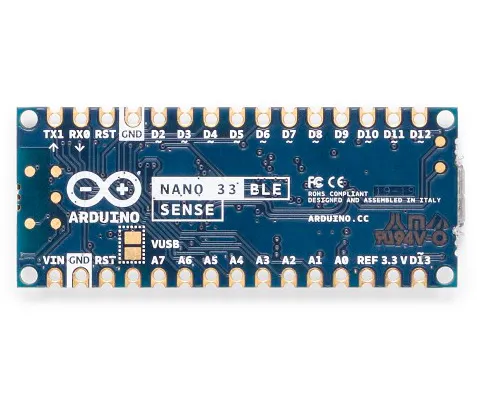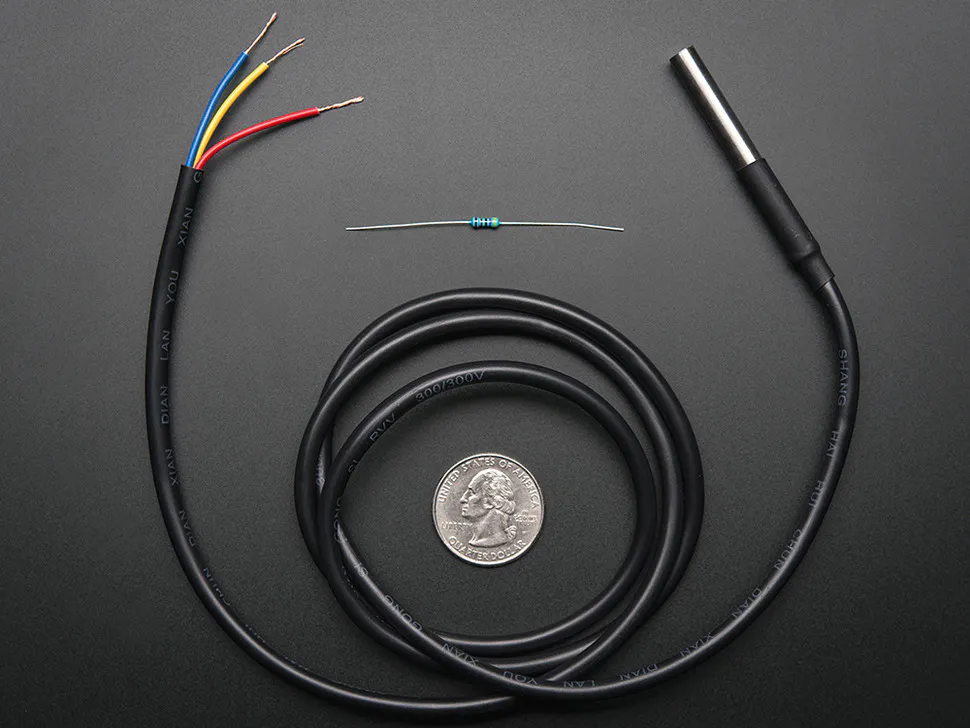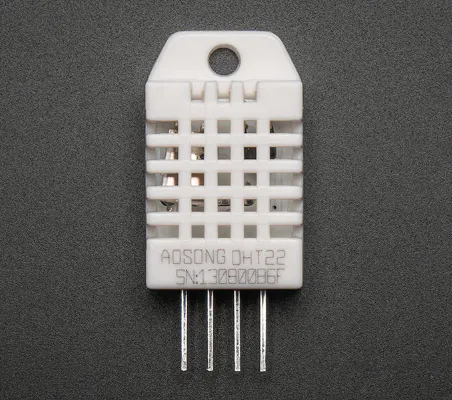Published

# HaBeeBee : BeeHive Monitoring System

Our HaBeeBee Monitoring System allows the managing and monitoring beehives making life easier for beekeepers.

IntermediateFull instructions provided5 days499## Things used in this project

### Hardware componentsArduino Nano 33 BLE Sense
×1
 Solar panel SOL2W
×1
 Seeed Studio Carte LiPo Rider Pro 106990008
×1
 Seeed Studio LoRa-E5
×1
 Battery Li-Ion 3,7V 1050 mAh
×1Adafruit Waterproof DS18B20 Digital temperature sensor
×2
 Seeed Studio HX711+strain gauge
×1×2
 lapel microphone
×1
 DFRobot SEN0291
×1

### Software apps and online servicesArduino IDE
 BeepUbidotsThe Things Network## Code

### Final Code

Arduino
```#include <DHT.h>

#define MAXIMWIRE_EXTERNAL_PULLUP
#include "HX711.h"
#include <Wire.h>
#include <MaximWire.h>
#include "DFRobot_INA219.h"

#define PIN_BUS 7

#define calibration_factor -22072.0

HX711 scale;

MaximWire::Bus bus(PIN_BUS);
MaximWire::DS18B20 device;

#define DHTPIN D9
#define DHTPIN1 D10

#define DHTTYPE DHT22
DHT dht(DHTPIN, DHTTYPE);
DHT dht1(DHTPIN1, DHTTYPE);

const float TensionMax =4.2;
const float TensionMin =3.3;
float masse=0.0;
static char recv_buf;
static bool is_exist = false;
static bool is_join = false;
static int led = 0;
int ret=0;
int delay_min = 1;
float tmp =20;
float hum=50;
float tmp1 =20;
float hum1=50;
float moyenne={0,0,0,0,0,0,0,0,0,0};
int flag=0;
short masse_n=0;
short flag_temp=0;

float temp={2,2};

#define T 64
int data={14, 30, 35, 34, 34, 40, 46, 45, 30, 4,  -26,  -48,  -55,  -49,  -37,
-28,  -24,  -22,  -13,  6,  32, 55, 65, 57, 38, 17, 1,  -6, -11,  -19,  -34,
-51,  -61,  -56,  -35,  -7, 18, 32, 35, 34, 35, 41, 46, 43, 26, -2, -31,  -50,
-55,  -47,  -35,  -27,  -24,  -21,  -10,  11, 37, 58, 64, 55, 34, 13, -1, -7};

int freqd={98,146,195,244,293,342,391,439,488,537};
int freqf={146,195,244,293,342,391,439,488,537,586};

int cpt=0;
static int at_send_check_response(char *p_ack, int timeout_ms, char *p_cmd, ...){
int ch;
int num = 0;
int index = 0;
int startMillis = 0;
memset(recv_buf, 0, sizeof(recv_buf));
Serial1.write(p_cmd);
//Serial.write(p_cmd);
dht.begin();
dht1.begin();
delay(200);
startMillis = millis();
do{
while (Serial1.available() > 0){

recv_buf[index++] = ch;
//Serial.write(ch);
delay(2);
}
} while (millis() - startMillis < timeout_ms);

if (strstr(recv_buf, p_ack) != NULL){return 1;}
else return 0;
}

void setup() {
// put your setup code here, to run once:
delay(10000);
//Serial.begin(9600);
Serial1.begin(9600);
pinMode(12,OUTPUT);
digitalWrite(D12,HIGH);
pinMode(LED_BUILTIN, OUTPUT);
digitalWrite(PIN_ENABLE_SENSORS_3V3, LOW);
digitalWrite(PIN_ENABLE_I2C_PULLUP, LOW);

scale.set_scale(calibration_factor); //This value is obtained by using the SparkFun_HX711_Calibration sketch
//scale.tare(); //Assuming there is no weight on the scale at start up, reset the scale to 0

//Serial.print("E5 LORAWAN TEST\r\n");
if(at_send_check_response("+AT: OK", 100, "AT\r\n")){
is_exist = true;
at_send_check_response("+ID: AppEui", 1000, "AT+ID\r\n");
at_send_check_response("+MODE: LWOTAA", 1000, "AT+MODE=LWOTAA\r\n");
at_send_check_response("+DR: EU868", 1000, "AT+DR=EU868\r\n");
at_send_check_response("+CH: NUM", 1000, "AT+CH=NUM,0-2\r\n");
at_send_check_response("+KEY: APPKEY", 1000, "AT+KEY=APPKEY,\"E2615C4277914656365B2A0F5F012047\"\r\n");
at_send_check_response("+ID: DEVEUI", 1000, "AT+ID=DEVEUI,\"ABCDEF123456789A\"\r\n");
at_send_check_response("+ID: APPEUI", 1000, "AT+ID=APPEUI,\"0000000000000000\"\r\n");
at_send_check_response("+CLASS: C", 1000, "AT+CLASS=A\r\n");
ret=at_send_check_response("+PORT: 9", 1000, "AT+PORT=9\r\n");
delay(200);
is_join = true;

}
else{
is_exist = false;
//Serial.print("No E5 module found.\r\n");
}

/*Config capteur intensité*/
/*while(!Serial);

Serial.println();
while(ina219.begin() != true) {
Serial.println("INA219 begin faild");
delay(2000);
}*/
ina219.begin();
delay(2000);
//Serial.println();
}

void loop() {
digitalWrite(D12,HIGH);
digitalWrite(LED_PWR, HIGH);
/* Poids, DHT:temperature et humidité*/
flag=0;
flag_temp=0;
delay(1000);
scale.power_up();
//masse=scale.get_units();
if (scale.get_units()-1<0)
flag=1;
delay(1000);
//Serial.println(tmp);
//Serial.println(hum);
if (tmp<0)
{
flag_temp|=1<<1;
tmp=abs(tmp);
}
if (tmp1<0)
{
flag_temp|=1<<4;
tmp1=abs(tmp1);
}
delay(5000);

/*FFT*/

int i = 0;
int tab[T];
for (i=0;i<T;i++){
delayMicroseconds(500);
}
unsigned long StartTime= micros();
float f=Q_FFT(tab,T,2000);
unsigned long CurrentTime= micros();
unsigned long ElapsedTime= CurrentTime - StartTime;

delay(1000);

/* Etat batterie */
float bat= a0*5/1523;

delay(1000);

/* Code intensité "lumineuse" */
float Power = ina219.getPower_mW();
//float Power=1;

float intensity=ina219.getCurrent_mA();

if(intensity<0)
intensity=0;

/*Intensité max pour environ 200mA*/

/*Temperature DS18B20 */
MaximWire::Discovery discovery = bus.Discover();
do {

temp[cpt] = device.GetTemperature<float>(bus);
device.Update(bus);
}
}
if (cpt==1)
cpt=0;
else
cpt++;
} while (discovery.HaveMore());

if (temp<0)
{
flag_temp|=1<<2;
temp=abs(temp);
}
if (temp<0)
{
flag_temp|=1<<3;
temp=abs(temp);
}
/*Serial.println(temp);
Serial.println(temp);
Serial.println(tmp1);
Serial.println(hum1);
Serial.print(scale.get_units(), 2); //scale.get_units() returns a float
Serial.println(" kg"); //You can change this to kg but you'll need to refactor the calibration_factor
Serial.print("Poids:");
Serial.println(masse);*/

if (is_exist){

int ret = 0;

if (is_join){

ret = at_send_check_response("+JOIN: Network joined", 12000, "AT+JOIN\r\n");

if (ret){
is_join = false;

/*Serial.println();
Serial.print("Network JOIN !\r\n\r\n");*/

}

else{
at_send_check_response("+ID: AppEui", 1000, "AT+ID\r\n");
/*Serial.println();
Serial.print("JOIN failed!\r\n\r\n");*/
delay(3000);
}
}
else{

char cmd;

for(int i=0;i<10;i++)
{
//Serial.println(moyenne[i]);
}
if (!flag)
{
sprintf(cmd, "AT+MSGHEX=%04X%04X%04X%04X%04X%04X%04X%04X%04X%04X%04X%04X%04X%04X%04X%04X%04X%04X%04X%04X\r\n",(short) ((tmp*100)/10),(short) hum*100,(short)((temp*100)/10),(short)((temp*100)/10),(short) (2*bat*100),(short)(ina219.getBusVoltage_V()*100),(short)moyenne,(short)moyenne,(short)moyenne,(short)moyenne,(short)moyenne,(short)moyenne,(short)moyenne,(short)moyenne,(short)moyenne,(short)moyenne,(short) ((tmp1*100)/10),(short) hum1*100,(short)((scale.get_units()-1)*100),flag_temp); /* Résolution de 0.1°C*/
digitalWrite(LED_PWR, LOW);
scale.power_down();
}
else
{
sprintf(cmd, "AT+MSGHEX=%04X%04X%04X%04X%04X%04X%04X%04X%04X%04X%04X%04X%04X%04X%04X%04X%04X%04X%04X%04X\r\n",(short) ((tmp*100)/10),(short) hum*100,(short)((temp*100)/10),(short)((temp*100)/10),(short) (2*bat*100),(short)(ina219.getBusVoltage_V()*100),(short)moyenne,(short)moyenne,(short)moyenne,(short)moyenne,(short)moyenne,(short)moyenne,(short)moyenne,(short)moyenne,(short)moyenne,(short)moyenne,(short) ((tmp1*100)/10),(short) hum1*100,masse_n,flag_temp); /* Résolution de 0.1°C*/
scale.power_down();
digitalWrite(LED_PWR, LOW);
}

}
}

else{delay(1000);}
delay(600000);

/* char* downlink = strstr(recv_buf, "\"");
if (delay_min < 1) delay_min = 1;
if (delay_min > 60) delay_min = 60;
delay(delay_min*60000);*/

}

float Q_FFT(int in[],int N,float Frequency)
{

unsigned int Pow2={1,2,4,8,16,32,64,128,256,512,1024,2048}; // declaring this as global array will save 1-2 ms of time

int a,c1,f,o,x;
byte check=0;
a=N;

for(int i=0;i<12;i++)                 //calculating the levels
{ if(Pow2[i]<=a){o=i;} }

int out_r[Pow2[o]]={};   //real part of transform
int out_im[Pow2[o]]={};  //imaginory part of transform

x=0;
for(int b=0;b<o;b++)                     // bit reversal
{
c1=Pow2[b];
f=Pow2[o]/(c1+c1);
for(int j=0;j<c1;j++)
{
x=x+1;
out_im[x]=out_im[j]+f;
}
}

for(int i=0;i<Pow2[o];i++)            // update input array as per bit reverse order
{
out_r[i]=in[out_im[i]];
out_im[i]=0;
}

int i10,i11,n1,tr,ti;
float e;
int c,s;
for(int i=0;i<o;i++)                                    //fft
{
i10=Pow2[i];              // overall values of sine/cosine
i11=Pow2[o]/Pow2[i+1];
e=360/Pow2[i+1];
e=0-e;
n1=0;

for(int j=0;j<i10;j++)
{
c=e*j;
while(c<0){c=c+360;}
while(c>360){c=c-360;}

n1=j;

for(int k=0;k<i11;k++)
{

if(c==0) { tr=out_r[i10+n1];
ti=out_im[i10+n1];}
else if(c==90){ tr= -out_im[i10+n1];
ti=out_r[i10+n1];}
else if(c==180){tr=-out_r[i10+n1];
ti=-out_im[i10+n1];}
else if(c==270){tr=out_im[i10+n1];
ti=-out_r[i10+n1];}
else if(c==360){tr=out_r[i10+n1];
ti=out_im[i10+n1];}
else if(c>0  && c<90)   {tr=out_r[i10+n1]-out_im[i10+n1];
ti=out_im[i10+n1]+out_r[i10+n1];}
else if(c>90  && c<180) {tr=-out_r[i10+n1]-out_im[i10+n1];
ti=-out_im[i10+n1]+out_r[i10+n1];}
else if(c>180 && c<270) {tr=-out_r[i10+n1]+out_im[i10+n1];
ti=-out_im[i10+n1]-out_r[i10+n1];}
else if(c>270 && c<360) {tr=out_r[i10+n1]+out_im[i10+n1];
ti=out_im[i10+n1]-out_r[i10+n1];}

out_r[n1+i10]=out_r[n1]-tr;
out_r[n1]=out_r[n1]+tr;
if(out_r[n1]>15000 || out_r[n1]<-15000){check=1;}

out_im[n1+i10]=out_im[n1]-ti;
out_im[n1]=out_im[n1]+ti;
if(out_im[n1]>15000 || out_im[n1]<-15000){check=1;}

n1=n1+i10+i10;
}
}

if(check==1){                                             // scale the matrics if value higher than 15000 to prevent varible from overloading
for(int i=0;i<Pow2[o];i++)
{
out_r[i]=out_r[i]/100;
out_im[i]=out_im[i]/100;
}
check=0;
}

}

//---> here onward out_r contains amplitude and our_in conntains frequency (Hz)
int fout,fm,fstp;
float fstep;
fstep=Frequency/N;
fstp=fstep;
fout=0;fm=0;
int cpt1=0;
int cptn=0;
for (int i=0;i<10;i++)
moyenne[i]=0;
for(int i=1;i<Pow2[o-1];i++)               // getting amplitude from compex number
{
if((out_r[i]>=0) && (out_im[i]>=0)){out_r[i]=out_r[i]+out_im[i];}
else if((out_r[i]<=0) && (out_im[i]<=0)){out_r[i]=-out_r[i]-out_im[i];}
else if((out_r[i]>=0) && (out_im[i]<=0)){out_r[i]=out_r[i]-out_im[i];}
else if((out_r[i]<=0) && (out_im[i]>=0)){out_r[i]=-out_r[i]+out_im[i];}
// to find peak sum of mod of real and imaginery part are considered to increase speed

out_im[i]=out_im[i-1]+fstp;
if (fout<out_r[i]){fm=i; fout=out_r[i];}
//Serial.print(out_im[i]);Serial.print("Hz");
//Serial.print("\t");                            // un comment to print freuency bin
//Serial.println(out_r[i]);
if (out_im[i]>=freqd[cpt1] && out_im[i]<=freqf[cpt1])
{
moyenne[cpt1]+=out_r[i];
cptn++;
}
else if (out_im[i]>=freqd)
{
if (cptn>0)
moyenne[cpt1]/=cptn;
cpt1++;
cptn=0;
}
}

}
```

## Credits

### Petitot Victor

0 projects • 0 followers

### Moufees Mohamed

0 projects • 0 followers